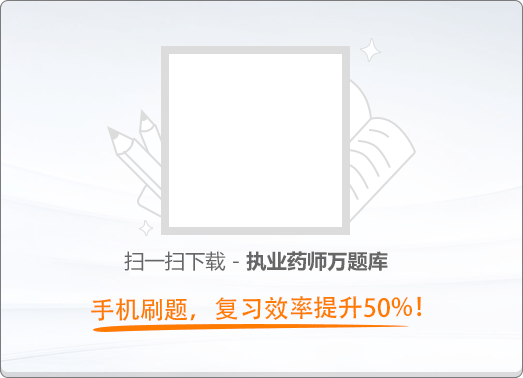150:00【新大纲】《药学专业知识（一）》历年真题试卷（二）

1
(单项选择题)

• A.

乳剂液滴的分散度大

• B.

乳剂中药物吸收快

• C.

乳剂的生物利用度高

• D.

一般W/O型乳剂专供静脉注射用

• E.

静脉注射乳剂注射后分布较快，有靶向性

• A
• B
• C
• D
• E

2
(单项选择题)

• A.

降低两相液体的表面张力

• B.

形成牢固的乳化膜

• C.

确定形成乳剂的类型

• D.

有适当的相比

• E.

加入反絮凝剂

• A
• B
• C
• D
• E

3
(单项选择题)

• A.

维生素A

• B.

维生素B1

• C.

维生素C

• D.

青霉素钠

• E.

硫酸链霉素

• A
• B
• C
• D
• E

7
(单项选择题)

• A.

产生最大效应所需剂量的一半

• B.

引起50%阳性反应（质反应）或50%最大效应（量反应）的浓度或剂量

• C.

一半动物产生毒性反应的剂量

• D.

产生等效反应所需剂量的一半

• E.

常用治疗量的一半

• A
• B
• C
• D
• E

8
(单项选择题)

• A.

一次性用药超过剂量

• B.

长期用药蓄积

• C.

病人属于过敏体质

• D.

病人肝功能或肾功能损害

• E.

可致畸胎、突变

• A
• B
• C
• D
• E

9
(单项选择题)

• A.

主动转运吸收

• B.

简单扩散吸收

• C.

易化扩散吸收

• D.

通过载体吸收

• E.

过滤方式吸收

• A
• B
• C
• D
• E

10
(单项选择题)

• A.

异丙肾上腺素

• B.

可待因

• C.

二羟丙茶碱

• D.

色甘酸钠

• E.

苯佐那酯

• A
• B
• C
• D
• E

11
(单项选择题)

• A.

性状

• B.

鉴别

• C.

检查

• D.

含量测定

• E.

类别

• A
• B
• C
• D
• E

12
(单项选择题)

• A.

不超过30℃

• B.

不超过20℃

• C.

避光并不超过30℃

• D.

避光并不超过20℃

• E.

2～10℃

• A
• B
• C
• D
• E

14
(单项选择题)

• A.

酚酞

• B.

淀粉

• C.

荧光黄

• D.

邻二氮菲

• E.

铬黑T

• A
• B
• C
• D
• E

16
(单项选择题)

• A.

用药剂量过大

• B.

用药时间过长

• C.

机体敏感性太高

• D.

组织器对药物亲和力过大

• E.

药物作用选择性低

• A
• B
• C
• D
• E

17
(单项选择题)

• A.

躯体依赖性

• B.

首剂现象

• C.

耐药性

• D.

耐受性

• E.

致敏性

• A
• B
• C
• D
• E

18
(单项选择题)

• A.

目录

• B.

凡例

• C.

正文

• D.

附录

• E.

索引

• A
• B
• C
• D
• E

19
(单项选择题)

• A.

表面活性剂对药物吸收有影响

• B.

在表面活性剂中，非离子表面活性剂的毒性最大

• C.

表面活性剂与蛋白质可发生相互作用

• D.

表面活性剂长期应用或高浓度使用可能出现皮肤或黏膜损伤

• E.

表面活性剂静脉注射的毒性大于口服

• A
• B
• C
• D
• E

20
(单项选择题)

• A.

咀嚼片是指含有碳酸氢钠和枸橼酸作为崩解剂的片剂

• B.

多层片是指含有碳酸氢钠和枸橼酸作为崩解剂的片剂

• C.

薄膜衣片是以丙烯酸树脂或蔗糖为主要包衣材料的片剂

• D.

口含片是专用于舌下的片剂

• E.

缓释片是指能够延长药物作用时间的片剂

• A
• B
• C
• D
• E

21
(单项选择题)

• A.

密闭

• B.

密封

• C.

严封

• D.

熔封

• E.

避光

• A
• B
• C
• D
• E

22
(单项选择题)

• A.

在稀盐酸溶液中，用亚硝酸钠滴定液滴定，永停法指示终点

• B.

在碳酸钠溶液中，用硝酸银滴定液滴定，电位法指示终点

• C.

用0.4%的氢氧化钠溶液溶解，在304nm处测定吸收度

• D.

用中性乙醇溶解，用氢氧化钠滴定液滴定，酚酞作指示剂

• E.

用冰醋酸溶解，用高氯酸滴定液滴定

• A
• B
• C
• D
• E

23
(单项选择题)

《中国药典》（2015年版）规定薄膜衣片的崩解时限为（　）。

• A.

5分钟内

• B.

15分钟内

• C.

30分钟内

• D.

60分钟内

• E.

120分钟内

• A
• B
• C
• D
• E

24
(单项选择题)

• A.

输液是指由静脉滴注输入体内的大剂量注射液

• B.

除无菌外还必须无热原

• C.

渗透压应为等渗或偏高渗

• D.

为保证无菌，需添加抑菌剂

• E.

澄明度应符合要求

• A
• B
• C
• D
• E

25
(单项选择题)

• A.

混悬粒子的沉降速度

• B.

微粒的荷电与水化

• C.

混悬剂中药物的降解

• D.

絮凝与反絮凝

• E.

结晶生长与转型

• A
• B
• C
• D
• E

26
(单项选择题)

• A.

泡腾剂

• B.

消泡剂

• C.

芳香剂

• D.

胶浆剂

• E.

甜味剂

• A
• B
• C
• D
• E

27
(单项选择题)

• A.

药物的降解速度与离子强度无关

• B.

液体制剂的辅料影响药物稳定性，而固体制剂的辅料不影响药物稳定性

• C.

药物的降解速度与溶剂无关

• D.

零级反应的速度与反应物浓度无关

• E.

经典恒温法适用于所有药物的稳定性研究

• A
• B
• C
• D
• E

28
(单项选择题)

• A.

防止其失活

• B.

减少在体内驻留时间

• C.

防止其挥发

• D.

减少其对胃肠道的刺激性

• E.

增加其溶解度

• A
• B
• C
• D
• E

29
(单项选择题)

• A.

载体材料为水溶性

• B.

载体材料对药物有抑晶性

• C.

载体材料提高了药物分子的再聚集性

• D.

载体材料提高了药物的可润湿性

• E.

载体材料保证了药物的高度分散性

• A
• B
• C
• D
• E

30
(单项选择题)

• A.

制成包衣小丸或包衣片剂

• B.

制成微囊

• C.

与高分子化合物生成难溶性盐或酯

• D.

制成不溶性骨架片

• E.

制成亲水凝肢骨架片

• A
• B
• C
• D
• E

31
(单项选择题)

• A.

被动靶向制剂

• B.

主动靶向制剂

• C.

物理靶向制剂

• D.

化学靶向制剂

• E.

物理化学靶向制剂

• A
• B
• C
• D
• E

32
(单项选择题)

• A.

静脉注射

• B.

腹腔注射

• C.

肌内注射

• D.

口服给药

• E.

肺部给药

• A
• B
• C
• D
• E

34
(单项选择题)

• A.

静脉注射

• B.

皮下注射

• C.

皮内注射

• D.

鞘内注射

• E.

腹腔注射

• A
• B
• C
• D
• E

35
(单项选择题)

• A.

药物剂型

• B.

药物制剂

• C.

药剂学

• D.

调剂学

• E.

方剂

• A
• B
• C
• D
• E

36
(单项选择题)

• A.

被动扩散的物质可由高浓度区向低浓度区转运，转运的速度为一级速度

• B.

促进扩散的转运速率低于被动扩散

• C.

主动转运借助于栽体进行，不需消耗能量

• D.

被动扩散会出现饱和现象

• E.

胞饮作用对于蛋白质和多肽的吸收不是十分重要

• A
• B
• C
• D
• E

37
(单项选择题)

• A.

泡腾栓剂

• B.

渗透泵栓剂

• C.

凝胶栓剂

• D.

双层栓剂

• E.

微囊栓剂

• A
• B
• C
• D
• E

38
(单项选择题)

• A.

肾上腺素

• B.

盐酸麻黄碱

• C.

去甲肾上腺素

• D.

异丙肾上腺素

• E.

多巴胺

• A
• B
• C
• D
• E

39
(单项选择题)• A.

A

• B.

B

• C.

C

• D.

D

• E.

E

• A
• B
• C
• D
• E

40
(单项选择题)• A.

A

• B.

B

• C.

C

• D.

D

• E.

E

• A
• B
• C
• D
• E